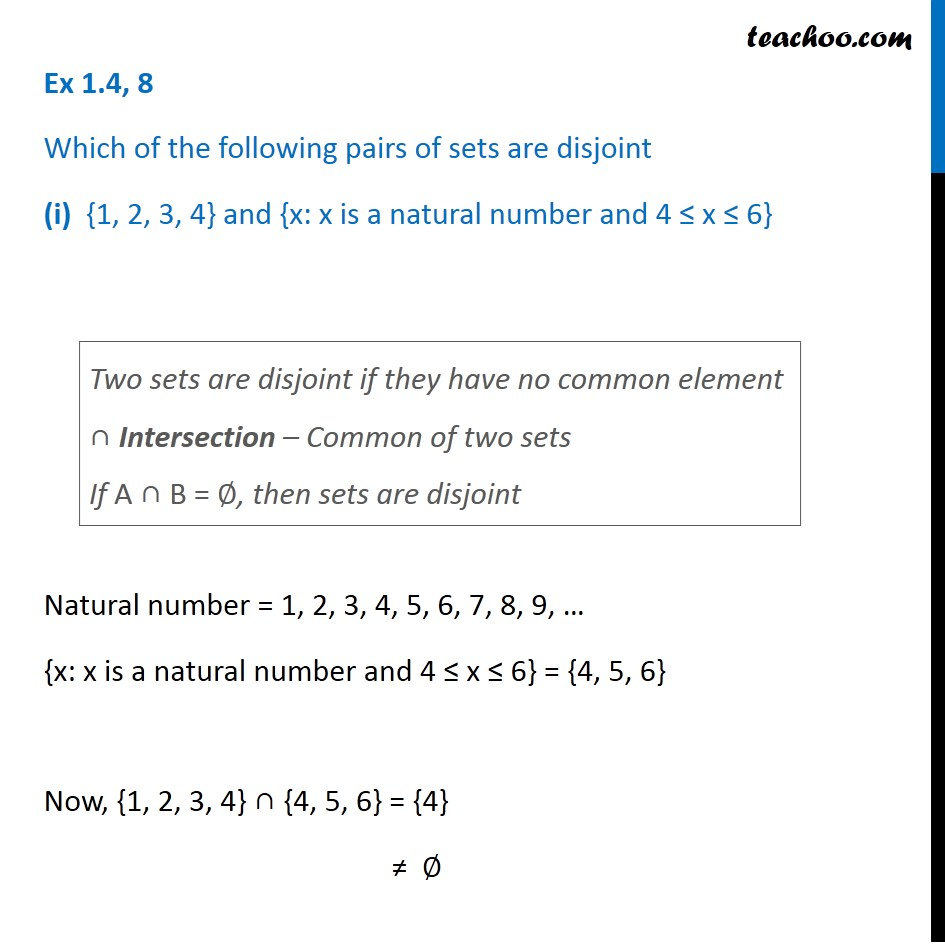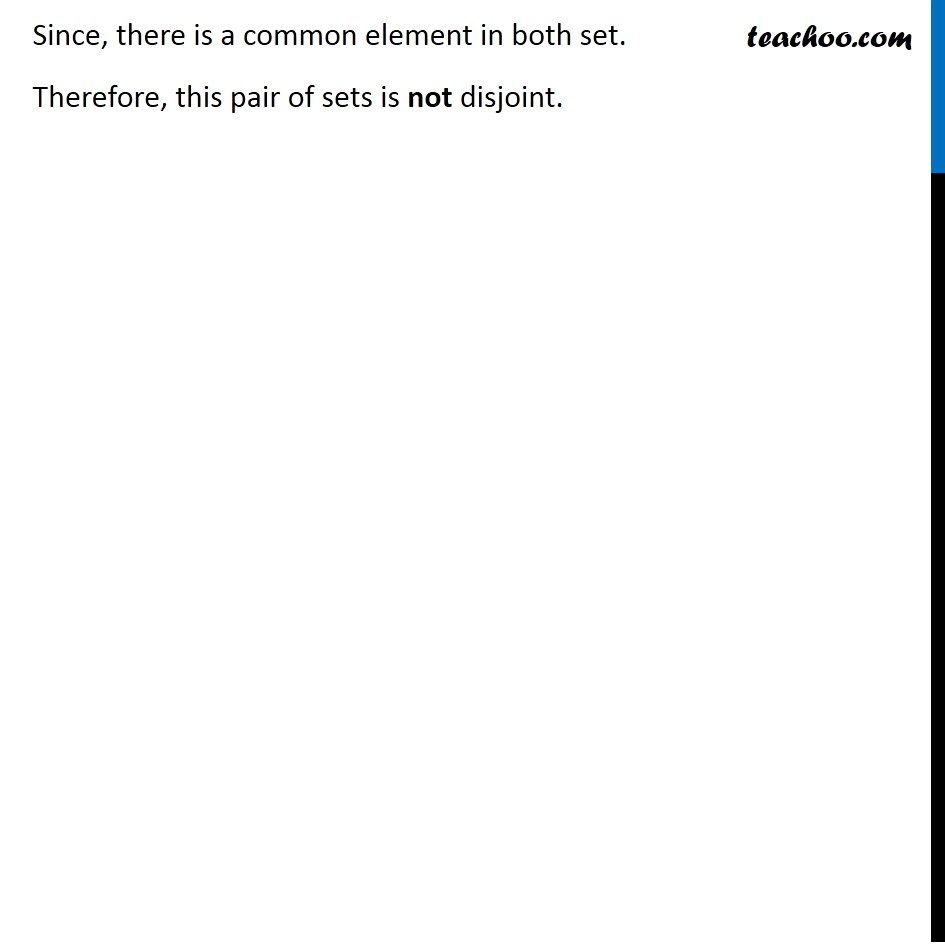1. Chapter 1 Class 11 Sets (Term 1)
2. Concept wise
3. Intersection of Sets

Transcript

Ex 1.4, 8 Which of the following pairs of sets are disjoint (i) {1, 2, 3, 4} and {x: x is a natural number and 4 ≤ x ≤ 6} Natural number = 1, 2, 3, 4, 5, 6, 7, 8, 9, … {x: x is a natural number and 4 ≤ x ≤ 6} = {4, 5, 6} Now, {1, 2, 3, 4} ∩ {4, 5, 6} = {4} ≠ ∅ Two sets are disjoint if they have no common element ∩ Intersection – Common of two sets If A ∩ B = ∅, then sets are disjoint Since, there is a common element in both set. Therefore, this pair of sets is not disjoint.